# 2 Axis Digital Bubble Level V1.0

A 2 axis level utilizing an MPU6050 (GY-521), Arduino Mega, 8X8 LED Matric (MAX7219) and 7 standard LEDs. Modular precision & fast response.

IntermediateShowcase (no instructions)9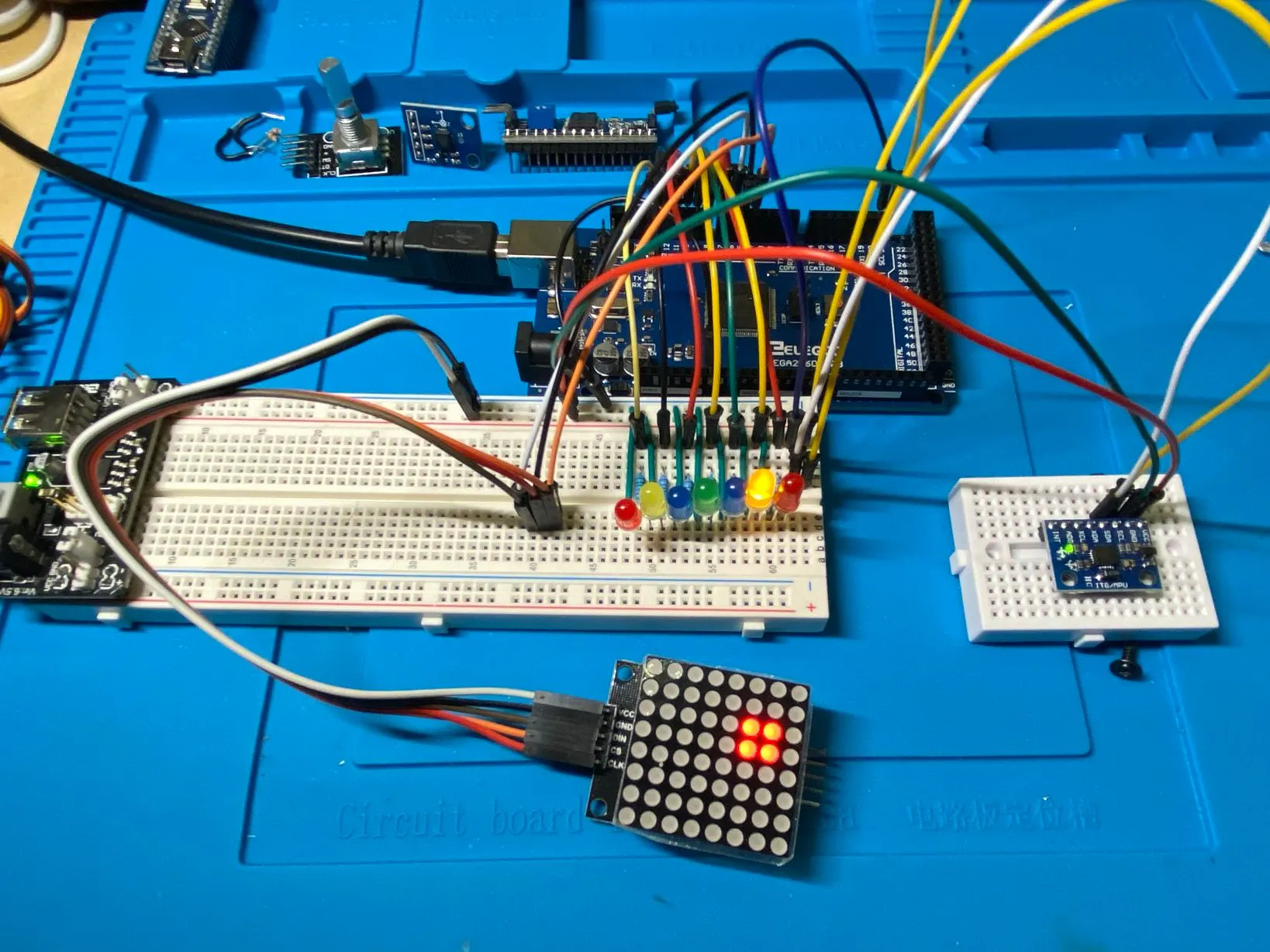## Things used in this project

### Hardware components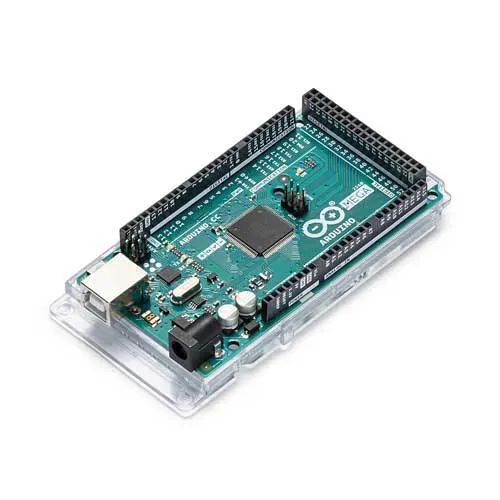Arduino Mega 2560 Could use UNO, maybe NANO
×1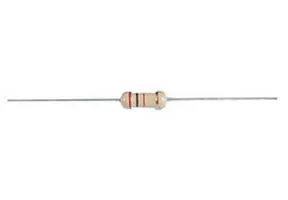Resistor 220 ohm
×7

### Hand tools and fabrication machines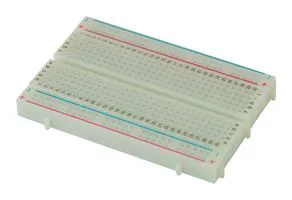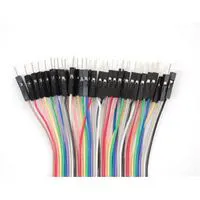Servo Motor, Premium Male/Male Jumper Wires

## Schematics

### 2Axis-Digital-Level-V1

Wiring Diagram for this project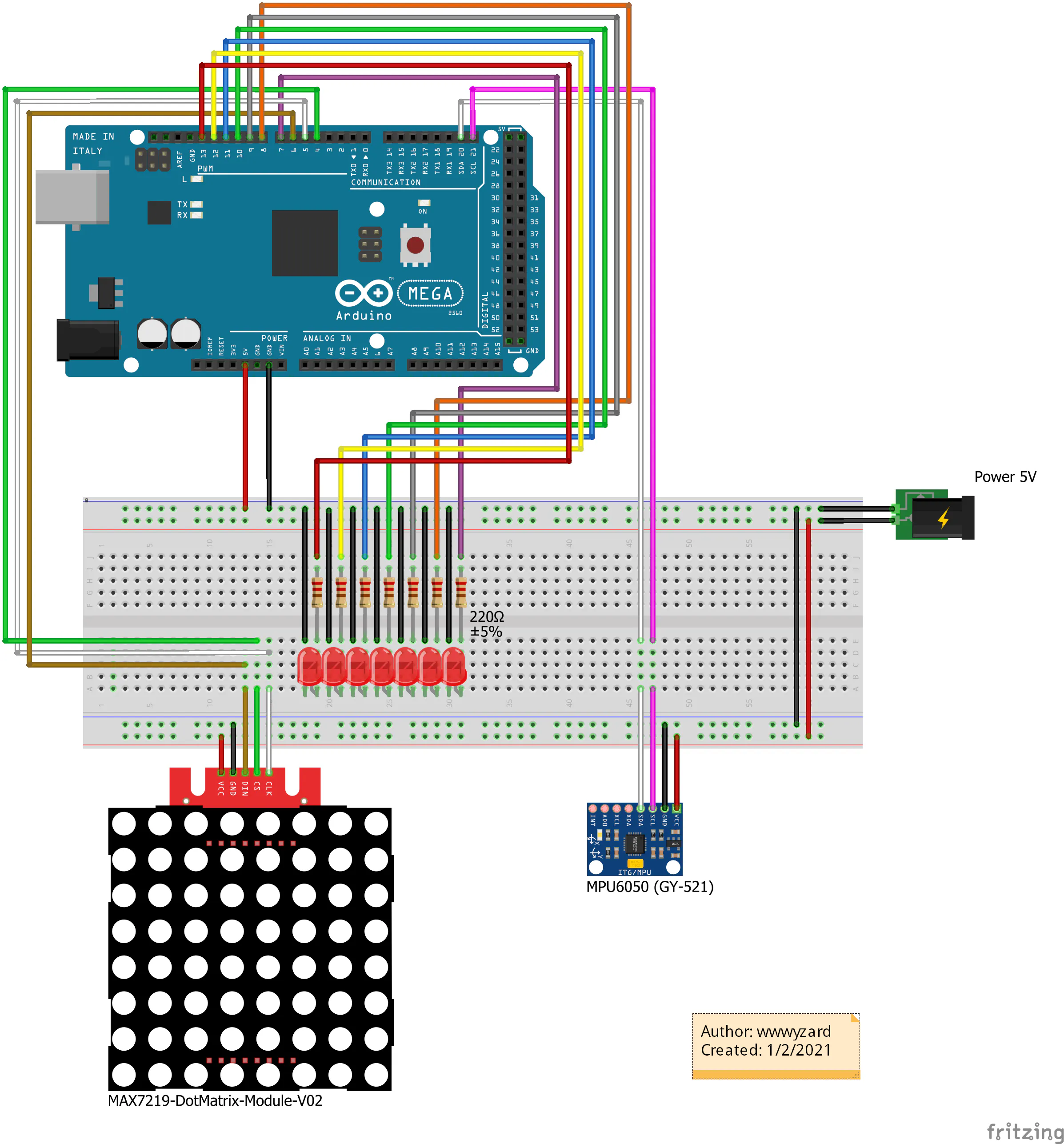## Code

### 2Axis-Digital-Level-V1

Arduino
Arduino Code for this project
```// Include Wire Library for I2C
#include <Wire.h>

// Include for 8X8 Dot Matrix
#include "LedControl.h"
LedControl lc = LedControl (6, 5, 4, 1);

//Tolerance
// 0-10 = Ultra Fast - Loose accuracy but averages ok
// 11-100 is more acurrate but slower as you scale up.
const int tolerance = 10;

//Precision
const float Low4 = -1.00;
const float Low3 = -0.75;
const float Low2 = -0.50;
const float Low1 = -0.25;
const float High1 = 0.25;
const float High2 = 0.50;
const float High3 = 0.75;
const float High4 = 01.00;

int led0 = 7;
int led1 = 8;
int led2 = 9;
int led3 = 10;
int led4 = 11;
int led5 = 12;
int led6 = 13;

// Vars for positioning later
int row;
int col;

// Display counter
int displaycount = 0;

//Variables for Gyroscope
int gyro_x, gyro_y, gyro_z;
long gyro_x_cal, gyro_y_cal, gyro_z_cal;
boolean set_gyro_angles;

long acc_x, acc_y, acc_z, acc_total_vector;
float angle_roll_acc, angle_pitch_acc;

float angle_pitch, angle_roll;
int angle_pitch_buffer, angle_roll_buffer;
float angle_pitch_output, angle_roll_output;

// Setup temp variable
int temp;

void setup() {

// the MAX72XX is in power-saving mode on startup,
// we have to do a wakeup call
lc.shutdown (0, false);

//Start I2C
Wire.begin();

// Set Board LEDs as outputs
pinMode(led0, OUTPUT);
pinMode(led1, OUTPUT);
pinMode(led2, OUTPUT);
pinMode(led3, OUTPUT);
pinMode(led4, OUTPUT);
pinMode(led5, OUTPUT);
pinMode(led6, OUTPUT);

//Setup the registers of the MPU-6050
setup_mpu_6050_registers();

//Read the raw acc and gyro data from the MPU-6050 1000 times
for (int cal_int = 0; cal_int < 1000 ; cal_int ++) {
//Add the gyro x offset to the gyro_x_cal variable
gyro_x_cal += gyro_x;
//Add the gyro y offset to the gyro_y_cal variable
gyro_y_cal += gyro_y;
//Add the gyro z offset to the gyro_z_cal variable
gyro_z_cal += gyro_z;
//Delay 3us to have 250Hz for-loop
delay(3);
}

// Divide all results by 1000 to get average offset
gyro_x_cal /= 1000;
gyro_y_cal /= 1000;
gyro_z_cal /= 1000;

// Start Serial Monitor
Serial.begin(115200);

}

void loop() {

// Get data from MPU-6050

//Subtract the offset values from the raw gyro values
gyro_x -= gyro_x_cal;
gyro_y -= gyro_y_cal;
gyro_z -= gyro_z_cal;

//Gyro angle calculations . Note 0.0000611 = 1 / (250Hz x 65.5)

//Calculate the traveled pitch angle and add this to the angle_pitch variable
angle_pitch += gyro_x * 0.0000611;
//Calculate the traveled roll angle and add this to the angle_roll variable
//0.000001066 = 0.0000611 * (3.142(PI) / 180degr) The Arduino sin function is in radians
angle_roll += gyro_y * 0.0000611;

//If the IMU has yawed transfer the roll angle to the pitch angle
angle_pitch += angle_roll * sin(gyro_z * 0.000001066);
//If the IMU has yawed transfer the pitch angle to the roll angle
angle_roll -= angle_pitch * sin(gyro_z * 0.000001066);

//Accelerometer angle calculations

//Calculate the total accelerometer vector
acc_total_vector = sqrt((acc_x * acc_x) + (acc_y * acc_y) + (acc_z * acc_z));

//57.296 = 1 / (3.142 / 180) The Arduino asin function is in radians
//Calculate the pitch angle
angle_pitch_acc = asin((float)acc_y / acc_total_vector) * 57.296;
//Calculate the roll angle
angle_roll_acc = asin((float)acc_x / acc_total_vector) * -57.296;

//Accelerometer calibration value for pitch
angle_pitch_acc -= 0.0;
//Accelerometer calibration value for roll
angle_roll_acc -= 0.0;

if (set_gyro_angles) {

//If the IMU has been running
//Correct the drift of the gyro pitch angle with the accelerometer pitch angle
angle_pitch = angle_pitch * 0.9996 + angle_pitch_acc * 0.0004;
//Correct the drift of the gyro roll angle with the accelerometer roll angle
angle_roll = angle_roll * 0.9996 + angle_roll_acc * 0.0004;
}
else {
//IMU has just started
//Set the gyro pitch angle equal to the accelerometer pitch angle
angle_pitch = angle_pitch_acc;
//Set the gyro roll angle equal to the accelerometer roll angle
angle_roll = angle_roll_acc;
//Set the IMU started flag
set_gyro_angles = true;
}

//To dampen the pitch and roll angles a complementary filter is used
//Take 90% of the output pitch value and add 10% of the raw pitch value
angle_pitch_output = angle_pitch_output * 0.9 + angle_pitch * 0.1;
//Take 90% of the output roll value and add 10% of the raw roll value
angle_roll_output = angle_roll_output * 0.9 + angle_roll * 0.1;
//Wait until the loop_timer reaches 4000us (250Hz) before starting the next loop

// Increment the display counter
displaycount = displaycount + 1;

if (displaycount > tolerance) {

// Print to Serial Monitor
Serial.print(" | Pitch: "); Serial.println(angle_pitch_output);
Serial.print(" | Roll: "); Serial.println(angle_roll_output);

// clear the 8X8 display
lc.clearDisplay (0);

// Get position for BoardLEDs and Matrix Row
// boardLights: 1 = On, 0 = Off,
if (angle_pitch_output < Low4) {
boardLights(1, 0, 0, 0, 0, 0, 0);
row = 6;
} else if ((angle_pitch_output > Low3) && (angle_pitch_output < Low2)) {
boardLights(0, 1, 0, 0, 0, 0, 0);
row = 5;
} else if ((angle_pitch_output > Low2) && (angle_pitch_output < Low1)) {
boardLights(0, 0, 1, 0, 0, 0, 0);
row = 4;
} else if ((angle_pitch_output > Low1) && (angle_pitch_output < High1)) {
boardLights(0, 0, 0, 1, 0, 0, 0);
row = 3;
} else if ((angle_pitch_output > High1) && (angle_pitch_output < High2)) {
boardLights(0, 0, 0, 0, 1, 0, 0);
row = 2;
} else if ((angle_pitch_output > High2) && (angle_pitch_output < High3)) {
boardLights(0, 0, 0, 0, 0, 1, 0);
row = 1;
} else if (angle_pitch_output > High4) {
boardLights(0, 0, 0, 0, 0, 0, 1);
row = 0;
}

//Get Col Position
if (angle_roll_output < Low4) {
col = 0;
} else if ((angle_roll_output > Low3) && (angle_roll_output < Low2)) {
col = 1;
} else if ((angle_roll_output > Low2) && (angle_roll_output < Low1)) {
col = 2;
} else if ((angle_roll_output > Low1) && (angle_roll_output < High1)) {
col = 3;
} else if ((angle_roll_output > High1) && (angle_roll_output < High2)) {
col = 4;
} else if ((angle_roll_output > High2) && (angle_roll_output < High3)) {
col = 5;
} else if (angle_roll_output > High4) {
col = 6;
}

// set the brightness to a medium values
lc.setIntensity (0, 8);

//Light Up the 4 LED quadrant of the position of row & col (x & y axis)
lc.setLed (0, col, row, true);
lc.setLed (0, col + 1, row, true);
lc.setLed (0, col, row + 1, true);
lc.setLed (0, col + 1, row + 1, true);

if ((row == 3) && (col == 3)) {
lc.setIntensity (0, 16);
lc.setRow (0, 0, B11111111);
for (int i = 1; i < 7; i++) {
lc.setRow (0, i, B10000001);
}
lc.setRow (0, 7, B11111111);
} else {
lc.setIntensity (0, 8);
lc.setRow (0, 3, B00011000);
lc.setRow (0, 4, B00011000);
}
displaycount = 0;
}
}

void boardLights(int pos0, int pos1, int pos2, int pos3, int pos4, int pos5, int pos6) {
digitalWrite(led0, pos0);
digitalWrite(led1, pos1);
digitalWrite(led2, pos2);
digitalWrite(led3, pos3);
digitalWrite(led4, pos4);
digitalWrite(led5, pos5);
digitalWrite(led6, pos6);
}

void setup_mpu_6050_registers() {

//Activate the MPU-6050

//Start communicating with the MPU-6050
Wire.beginTransmission(0x68);
//Send the requested starting register
Wire.write(0x6B);
//Set the requested starting register
Wire.write(0x00);
//End the transmission
Wire.endTransmission();

//Configure the accelerometer (+/-8g)

//Start communicating with the MPU-6050
Wire.beginTransmission(0x68);
//Send the requested starting register
Wire.write(0x1C);
//Set the requested starting register
Wire.write(0x10);
//End the transmission
Wire.endTransmission();

//Configure the gyro (500dps full scale)

//Start communicating with the MPU-6050
Wire.beginTransmission(0x68);
//Send the requested starting register
Wire.write(0x1B);
//Set the requested starting register
Wire.write(0x08);
//End the transmission
Wire.endTransmission();

}

//Read the raw gyro and accelerometer data

//Start communicating with the MPU-6050
Wire.beginTransmission(0x68);
//Send the requested starting register
Wire.write(0x3B);
//End the transmission
Wire.endTransmission();
//Request 14 bytes from the MPU-6050
Wire.requestFrom(0x68, 14);
//Wait until all the bytes are received
while (Wire.available() < 14);

//Following statements left shift 8 bits, then bitwise OR.
//Turns two 8-bit values into one 16-bit value